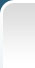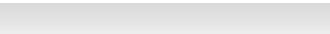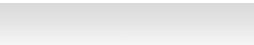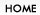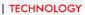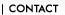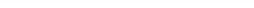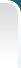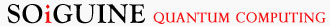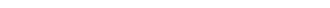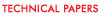Publications on Quantum Computing

• Soiguine A
"Explaining Some Weird Quantum Mechanical Features in Geometric Algebra Formalism".
• Soiguine A.
The Geometric Algebra Lift of Qubits and Beyond.
LAMBERT Academic Publishing, 2020.
• Soiguine A
"Scattering of Geometric Algebra Wave Functions and Collaps in Measurements".
• Soiguine A.
"States Instantly Spreading through Space and Forward and Backward in Time".
• Soiguine A.
"Sorry, Albert!".

In The XVII Conference on FAMEMS and III Workshop on Hilbert's Sixth Problem, Krasnoyarsk, Siberia, Russia,2018, pp.70-77
• Soiguine A.
"State/observable interactions using basic geometric algebra solutions of the Maxwell equation".
• Soiguine A.
"The geometric algebra lift of qubits via basic solutions of Maxwell equation".
• Soiguine A
"Polarizations as states and their evolution in geometric algebra terms with variable complex plane".
• Soiguine A.
"Quantum Computing with Variable Complex Plane. Light Beam Guide Implementation".
• Soiguine A.
"Anyons in three dimensions with geometric algebra".
• Soiguine A.
"Derivation of the Schrodinger equation in geometric algebra".
• Soiguine A.
Geometric Phase in Geometric Algebra Qubit Formalism.
LAMBERT Academic Publishing, 2015.
• Soiguine A.
"Geometric phase in G+3 quantum state evolution".
• Soiguine A.
"Quantum state evolution in C2 and G+3".
• Soiguine A.
"Geometric algebra, qubits, geometric evolution, and all that".
• Soiguine A.
"What quantum state really is?".
• Soiguine A.
"A tossed coin as quantum mechanical object".
• Soiguine A.
"Complex Conjugation - Relative to What?".

In Clifford Algebras with Numeric and Symbolic Computations. R.Ablamovicz, P.Lounesto and J.M. Parra eds., Birkhouser (Boston), 1996, pp. 285-294.
• Soiguine A..
Methods of Vector Algebra in Applied Problems.
Naval Academy Publ., Russia, 1990.

(Book on Clifford Algebra methods in physics and mathematics)